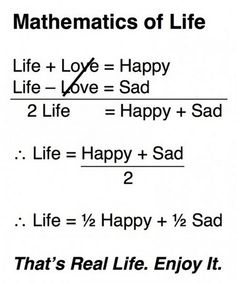## Spring Forward Into More Math! March 9-13, 2020

Math ….  a place to ponder deep and unique questions…..6th Grade Math – Mrs. Evans

Our class continues with Chapter 8, learning to solve equations. Students have to opportunity to extend our learning into two step equations if they are comfortable and confident with one step equation solutions!  We will also investigate inequalities; how they are evaluated, how to write them and how to graph them.  Student and families can use algebra to solve this problem.  Let me know if you have the answer!7th Grade Math – Mrs. Evans and Mrs. VonFeldt

This week we are still studying geometry as we investigate surface area of pyramids and  prisms of all types. Below are some prisms that we are working with in class.We will use a number of different methods to calculate the surface area, like nets and formulas.  Then we will find the volume of these same prisms.  Here is a great Math Antics video for those wanting a little more help understanding volume:

Algebra – Mrs. VonFeldt

We will finish up the chapter on exponents and exponential growth with a test on Tuesday. In Chapter 8, we will simplify, multiply, and factor polynomial expressions. Most of the problems will involve binomials, which are expressions with two terms. These skills will become very important as we continue our study of quadratic equations and graphs.

## Marching on in Math! March 2-6, 2020

Happy March!                                                                      This picture shows the importance of knowing math in school zones!6th Grade Math – Mrs. Evans

Our class has continued the study of algebra as we work in Chapter 8.  This chapter addresses equations and inequalities.  This week we will be using algebra to solve one step equations involving addition, subtraction, multiplication and division.  We have begun to work independently so that students can work to their speed and level.  Here is what it looks like to apply the principles we are learning this week to the mathematics of life:7th Grade Math – Mrs. Evans and Mrs. VonFeldt

This week we will continue the study of geometry as we investigate the area of basic shapes like circles, triangles, parallelograms and trapezoids.  Using those formulas we will be able to find the area of composite figures.  We will find it simple to determine the area of figures like the one below:As we finish the week, we will begin to consider 3 dimensional figures (prisms) and how we can find their surface areas.

Algebra – Mrs. VonFeldt

We are continuing to simplify exponential expressions using the properties of exponents. Students have explored all types of exponents including zero, negative, and fractional powers. This week we will look at exponential growth and decay and see how it is useful when considering interest rates or loan rates, something everyone will need to know! The Chapter 7 Test will be next Tuesday.